Chapter 20

As we know, the Quantum Hall effect can be used to define the impedance unit. There are two kinds of Quantum Hall effect: Integer Quantum Hall effect and Fractional Quantum Hall effect. The integer Quantum Hall effect can be regarded as a special case of Fractional Quantum Hall effect.

For the Fractional Quantum Hall effect, in which the two-dimensional electron gas within extremely low temperature and high magnetic field perpendicular to current, the Hall Resistance has the following equation: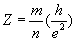(20.1)

In which m and n are the integers.

As we know, the electron has electric charge and magnetic charge, the magnetic charge distributes along with the electron spin directional, and the electron spin will align with the external magnetic field. The one side of the two dimensional electron gases will have positive magnetic charge distribution; the other side of the two dimensional electron gases has negative magnetic charge distribution.

Let us make an assumption that
the characteristic impedance is the ratio of magnetic charge and electric charge, then: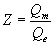(20.2)

As we know, the electron is the smallest unit of electron gas, the ‘e’ is the electric charge unit of the electron, and ‘g’ is the magnetic charge unit of an electron.  Thus, we have: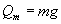(20.3)

And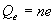(20.4)

Both ‘m’ and ‘n’ are integers.

Then we have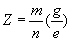(20.5)

As we know: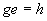(20.6)

Thus, we have:(20.7)

And so we get the result of the Fractional Quantum Hall effect.

When the ratio (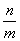) becomes the integer, the Quantum Hall effect is the Integer Quantum Hall effect. The Integer Quantum Hall effect is the special case of the fractional quantum hall effect.